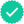#### Related questions

Question

A confidence interval for a population mean has a margin of error of 0.047.

a. Determine the length of the confidence interval.
b. If the sample mean is 0.205, obtain the confidence interval.
c. Construct a graph that illustrates your results.

Expert SolutionKnowledge Booster••